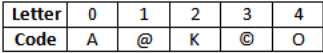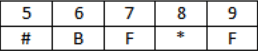Alphanumeric Series MCQ - 2

# Alphanumeric Series MCQ - 2 - Banking Exams

Test Description

## 12 Questions MCQ Test - Alphanumeric Series MCQ - 2

Alphanumeric Series MCQ - 2 for Banking Exams 2023 is part of Banking Exams preparation. The Alphanumeric Series MCQ - 2 questions and answers have been prepared according to the Banking Exams exam syllabus.The Alphanumeric Series MCQ - 2 MCQs are made for Banking Exams 2023 Exam. Find important definitions, questions, notes, meanings, examples, exercises, MCQs and online tests for Alphanumeric Series MCQ - 2 below.
Solutions of Alphanumeric Series MCQ - 2 questions in English are available as part of our course for Banking Exams & Alphanumeric Series MCQ - 2 solutions in Hindi for Banking Exams course. Download more important topics, notes, lectures and mock test series for Banking Exams Exam by signing up for free. Attempt Alphanumeric Series MCQ - 2 | 30 questions in 15 minutes | Mock test for Banking Exams preparation | Free important questions MCQ to study for Banking Exams Exam | Download free PDF with solutions
 1 Crore+ students have signed up on EduRev. Have you?
Alphanumeric Series MCQ - 2 - Question 1

### Directions (1-5): In each question below is given a group of digits followed by four combinations of digits/symbols given in (A), (B), (C) and (D). You have to find out which of the combinations correctly represents the group of digits based on the rules and mark the answer accordingly. If none of the combinations correctly represents the group of letters, mark (E) as your answer. (i) If the first digit is odd and last is even, then interchange the codes for both digits (ii) If there is a digit which is getting repeated in the given number, then code that digit as the code of 5 (iii) If the third digit is followed by an even number and is preceded by an odd number, then skip its code. (iv) If the middle digit is odd then interchange the codes of middle digit and last number. (v) 0 is neither odd nor evenQ. 3809125

Detailed Solution for Alphanumeric Series MCQ - 2 - Question 1

The given number satisfies rule (iv)

Alphanumeric Series MCQ - 2 - Question 2

### (i) If the first digit is odd and last is even, then interchange the codes for both digits (ii) If there is a digit which is getting repeated in the given number, then code that digit as the code of 5 (iii) If the third digit is followed by an even number and is preceded by an odd number, then skip its code. (iv) If the middle digit is odd then interchange the codes of middle digit and last number. (v) 0 is neither odd nor evenQ. 4302718

Detailed Solution for Alphanumeric Series MCQ - 2 - Question 2

The given number satisfies rule (iii)

Alphanumeric Series MCQ - 2 - Question 3

### Directions (6-10): Study the following arrangement and answer questions given: B 6 U & R 1 P 5 # F D @ I 2 Q 8 © K M % W 3 E A 4 * H 9 T Q. If all the numbers are dropped from the arrangement, then which of the following is the 6th letter to the right of the 20th letter from the right end?

Detailed Solution for Alphanumeric Series MCQ - 2 - Question 3

The series becomes: B U & R P # F D @ I Q © K M % W E A * H T

Alphanumeric Series MCQ - 2 - Question 4

Study the following arrangement and answer questions given:

B 6 U & R 1 P 5 # F D @ I 2 Q 8 © K M % W 3 E A 4 * H 9 T

Q. Complete the series: F@# 28I K%© ?

Alphanumeric Series MCQ - 2 - Question 5

Study the following arrangement and answer questions given:

B 6 U & R 1 P 5 # F D @ I 2 Q 8 © K M % W 3 E A 4 * H 9 T

Q. How many such consonants are there each of which is immediately preceded by a number?

Detailed Solution for Alphanumeric Series MCQ - 2 - Question 5

B 6 U & R 1 P 5 # F D @ I 2 Q 8 © K M % W 3 E A 4 * H 9 T

P, Q and T

Alphanumeric Series MCQ - 2 - Question 6

Directions (11-15): Study the following arrangement and answer questions given:

B L \$ 4 # G A 8 P X Y 7 % β N * S 2 @ D M = 9 * 5 C F 6

Q. How many such numbers are there which are immediately followed by a symbol and immediately preceded by letter?

Detailed Solution for Alphanumeric Series MCQ - 2 - Question 6

Y 7 %, S 2 @

Alphanumeric Series MCQ - 2 - Question 7

Study the following arrangement and answer questions given:

B L \$ 4 # G A 8 P X Y 7 % β N * S 2 @ D M = 9 * 5 C F 6

Q. If all the symbols are dropped from the above arrangement, then which is ninth from the right end?

Detailed Solution for Alphanumeric Series MCQ - 2 - Question 7

The series become:
B L 4 G A 8 P X Y 7 N S 2 D M 9 5 C F 6

Alphanumeric Series MCQ - 2 - Question 8

Study the following arrangement and answer questions given:

E 3 * L J 8 # R 1 4 % S A δ 6 K 9 M \$ X 7 β B Z @ 2 L

Q. How many such numbers are there in the above arrangement each of which is immediately preceded by a symbol and immediately followed by a letter ?

Detailed Solution for Alphanumeric Series MCQ - 2 - Question 8

δ 6 K, @ 2 L

Alphanumeric Series MCQ - 2 - Question 9

Study the following arrangement and answer questions given:

E 3 * L J 8 # R 1 4 % S A δ 6 K 9 M \$ X 7 β B Z @ 2 L

Q. Four of the five are alike in a certain way based on arrangement, which does not belong to the group?

Alphanumeric Series MCQ - 2 - Question 10

Directions: Study the following numbers carefully and answer the questions given beside:
867     495     736     592     619

Q. If all the digits are to be arranged in increasing order from left to right within the number then what will be the difference between the highest number and the lowest number thus obtained?

Detailed Solution for Alphanumeric Series MCQ - 2 - Question 10

The given sequence:

867     495     736     592     619

Arranging all the digits in increasing order from left to right within the number, we get:

678     459     367     259     169

Highest number = 678

Lowest number = 169

Required Difference = 678 – 169 = 509

Alphanumeric Series MCQ - 2 - Question 11

Directions: Study the following numbers carefully and answer the questions given beside:
867     495     736     592     619

Q.  If 1 is subtracted from each odd digit of each of the numbers first and then each perfect square digit is changed into 5, what is the sum of the least and the greatest number in the sequence thus obtained?

Detailed Solution for Alphanumeric Series MCQ - 2 - Question 11

The given sequence:

867     495     736     592     619

Subtracting 1 from each even digit of each of the numbers:

866     484     626     482     608

Changing every perfect square digit into 5, we get:

866     585     626     582     608

The sum of the least and the greatest number:

582 + 866 = 1448

Alphanumeric Series MCQ - 2 - Question 12

Study the following arrangement and answer questions given:

P  7  @  I  R  W  3  9  T  E  \$  4  H  D  5  ©  M  A  2  %  B  *  8  !  U  Q  N  1  F  6  #  K  V

Q. How many such numbers are there in the above arrangement, each of which is immediately preceded by a symbol and immediately followed by a consonant?

Information about Alphanumeric Series MCQ - 2 Page
In this test you can find the Exam questions for Alphanumeric Series MCQ - 2 solved & explained in the simplest way possible. Besides giving Questions and answers for Alphanumeric Series MCQ - 2, EduRev gives you an ample number of Online tests for practice(Scan QR code)